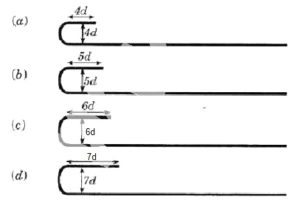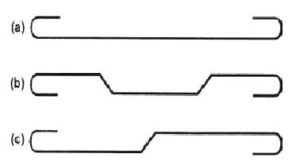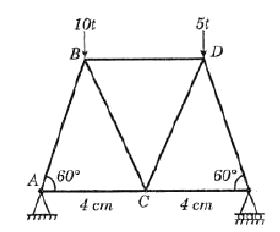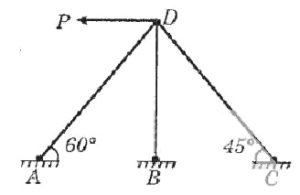Home / Civil / SSC Junior Engineer Exam Paper 2018 Shift-2 (Civil Engineering)

# SSC Junior Engineer Exam Paper 2018 Shift-2 (Civil Engineering)

ssc je civil

SSC Junior Engineer Exam Paper – 2018 “held on 22 January 2018” Evening Shift (Civil Engineering)

Q 1: The defect that is caused by falling of rain water on the hot surfaces of the bricks is known as______.
Options:
1) Bloating
2) chuffs
3) Cracks
4) lamination

Q 2: Which of the following property of bitumen is related to the Pensky-Marten test?
Options:
1) Ductility
2) Softening point
3) Flash and fire point
4) Viscosity

Q 3: The defect in timber that arises due to the swelling caused by growth of layers of sap wood over the wounds after branch is cut off is called as_______.
Options:
1) checks
2) knots
3) shakes
4) rind gall

Q 4: Which of the following is the measure of ease with which the wood may split?
Options:
1) Cleavability
2) Shearing strength
3) Stiffness
4) Toughness

Q 5: The get space ratio of a concrete sample is given as 0.589.
What is the theoretical strength (N/mm2) of that concrete sample ?
Options:
1) 49.04

2) 65.71
3) 104.03
4) 116.8

Q 6: Which of the following represents the CORRECT expression for maturity (M) of the concrete sample?
Options:
1)2)3)4)Answer:Q 7: The aggregate which is obtained from the seashore or rivers and produces minimum voids in the concrete is known as_______.
Options:
1) angular aggregates
2) flaky aggregates
3) irregular aggregates
4) rounded aggregates

Q 8: The detachment of the paint film from the surface is known as _____.
Options:
1) chalking
2) cracking
3) flaking
4) wrinkling

Q 9: Which of the following is the homogeneous solution of resins in the alcohol?
Options:
1) Distemper
2) Enamel  paint
3) Plastic paint
4) Varnish

Q 10: In the softening point test of the bitumen with the help of ring and ball apparatus, what is the diametre (cm) of the steel ball?
Options:
1) 0.35
2) 0.65
3) 0.95
4) 1.25

Q 11: Which of the following is the CORRECT statement for length of the short wall, as one move from earthwork to brick work in super structure in long and short wall method?
Options:
1) Its value decreases
2) Its value depends upon the length of the wall.
3) Its value increases.
4) Its value remains same.

Q 12: Which of the following is the unit of measurement for rivets?
Options:
1) Bags
2) Cubic meter
3) Numbers
4) Quintal

Q 13: Which of the following statement is CORRECT for units of measurement?
Options:
1) Bands of specified width are measured in running meter.
2) Work consists of the linear measurement and is measured in square meter.
3) Single units are measured in meter.
4) Work consists of areal surface and is measured in cubic meter.
Answer: Work consists of areal surface and is measured in cubic meter.

Q 14: Which of the following area is NOT included in the plinth area of the building?
Options:
1) Area of the lofts.
2) Area of Barsati at terrace level.
3) Area of walls at floor level.
4) Porches of non-cantilever type.

Q 15: Which of the following multiplying factor is used for the estimation of lead for Cartze tracks?
Options:
1) 0.8
2) 1
3) 1.1
4) 1.2

Q 16: Threading in the iron is measured in _____.
Options:
1) centimeter
2) kilogram
3) number
4) square centimeter

Q 17: Calculate the cost (Rs.) of 100 mm thick brick lining of a septic tank of size 5 m x 3 m x 1.5 m, if the rate of lining is Rs. 200 per square meter.
Options:
1) 4500
2) 4800
3) 5400
4) 7800

Q 18: Calculate the annual percentage depreciation of a machine using the constant percentage method, if purchasing cost is Rs. 12,000 and scrap value is Rs 3,000 and the life of the machine is 8 years.
Options:
1) 9.37
2) 16
3) 26.67
4) 33.33

Q 19: The number of the bricks delivered by an unskilled labour to a distance of 10 m in a working day is approximately _____.
Options:
1) 1500
2) 2200
3) 4200
4) 5500

Q 20: Calculate the quantity (cubic meter) of fine aggregate required for construction of a circular water tank of 3.5 m diameter and 5 m height, if M 25 cement concrete is used.
Options:
1) 12
2) 18.5
3) 37
4) 48

Q 21: Which type of error is represented by a closed traverse, if the algebraic sum of latitude of all the lines is zero?
Options:
1) Compensating error
2) Negative error
3) No error
4) Positive error

Q 22: The difference between the fore bearing and back bearing for any station is equal to _____.
Options:
1) external angle
2) either external or internal angle
3) internal angle
4) right angle
Answer: either external or internal angle

Q 23: Which of the following statement is CORRECT when the theodolite is properly adjusted?
Options:
1) Horizontal line passes through the centre of the horizontal circle.
2) Tangent to plate bubble must be perpendicular to horizontal axis.
3) Tangent to plate bubble must be perpendicular to vertical axis.
4) Vertical line passes through the centre of the vertical circle.
Answer: Tangent to plate bubble must be perpendicular to vertical axis.

Q 24: Which of the following test is used to make the horizontal axis perpendicular to the vertical axis?
Options:
1) Azimuth test
2) Cross hair ring test
3) Spire test
4) Vertical arc test

Q 25: For more precise prediction of the terrain relief, the contour interval should_____.
Options:
1) decrease continuously
2) increase continuously
3) be larger
4) be smaller
[sociallocker]
Q 26: The areas included by contour lines for a proposed dam are given as,

 Contour (m) 410 420 430 440 450 Area (hectares) 205 120 145 95 135

Options:
1) 42000000
2) 53000000
3) 70000000
4) 80000000

Q 27: Which of the following statement is CORRECT for estimating the corrected area from a map of shrunk factor ‘F’?
Options:
1) It is directly proportional to F.
2) It is directly proportional to square of F.
3) It is inversely proportional to F.
4) It is inversely proportional to square of F
Answer: It is inversely proportional to square of F

Q 28: Which of the following leveling method is used to determine the difference of elevation of two points that are quite apart?
Options:
1) Check leveling
2) Fly leveling
3) Reciprocal leveling
4) Simple leveling

Q 29: A level is set on a station at a distance of 500 m from point A and 800 m from point B. The staff readings on the staffs kept at point A and B are 1.55 m and 1.95 m respectively. Calculate the true difference in elevation of the point A and B.
Options:
1) 0.348
2) 0.374
3) 0.4
4) 0.426

Q 30: Which of the following method estimates the best volume of earthwork of an irregular embankment?
Options:
1) Average ordinate method
2) Mid-ordinate method
3) Simpson’s method
4) Trapezoidal method

Q 31: Which of the following represents the void ratio of soil sample whose porosity is 0.452?
Options:
1) 0.264
2) 0.561
3) 0.729
4) 0.825

Q 32: The moisture content in a soil sample is 18.2% and specific gravity of the soil particles is given by 2.65. What is the void ratio for sample, if the soil is fully saturated?
Options:
1) 0.157
2) 0.291
3) 0.482
4) 0.634

Q 33: If the degree of saturation of soil is given by 67.89%, what is the percentage of air content of the soil?
Options:
1) 10.5
2) 20.25
3) 32.11
4) 40.43

Q 34: Which of the following expression represent the relative compaction of soil, where variables have their standard meanings?
Options:
1)2)3)4) None of these
Answer:Q 35: The type of fluid in which flow and fluid properties does not change with time at any given location, is known as______.
Options:
1) non-uniform flow
2) rotational flow

Q 36: For a flow, the velocity components are given by u = (λxy2 – x3y2) and v = (x2y3 – 3y3). What is the value of λ for the possible flow field which includes steady incompressible flow ?
Options:
1) 3
2) 5
3) 7
4) 9

Q 37: What is the value of angle (degree) between stream lines and equipotential lines at the point of intersection in the flow net?
Options:
1) 0
2) 45
3) 60
4) 90

Q 38: What is the cross-sectional area (sq. m) of the channel, if its hydraulic radius and wetted perimeter is given as 300 cm and 860 cm respectively?
Options:
1) 10.5
2) 15.6
3) 25.8
4) 32.4

Q 39: The flow in a channel is laminar and Reynolds number is given by 1200. What is the friction factor for the channel?
Options:
1) 0.001
2) 0.008
3) 0.023
4) 0.053

Q 40: A circular pipe of diameter 0.5 m carries the discharge of 50 liters/s. The head loss due to friction in pipe is 0.15 m and friction factor for the pipe is given as 0.01. What is the length (m) of pipe?
Options:
1) 1150
2) 1860
3) 2263
4) 2785

Q 41: The Froude number for a hydraulic jump is 3.2, the type of jump is________.
Options:
1) oscillating jump
3) strong jump
4) weak jump

Q 42: At a certain point, the absolute pressure and atmospheric pressure is given by 850 mm of Hg and 700 mm of Hg respectively. What is the value of gauge pressure (mm of Hg) at that point?
Options:
1) 50
2) 100
3) 150
4) 200

Q 43: The head produced by a liquid of relative density 1.62 is equal to the pressure of 250 kPa. What is the head (m) produced by the liquid?
Options:
1) 5.04
2) 7.53
3) 15.73
4) 25.21

Q 44: Which of the following expression represents the  energy dissipated in the hydraulic jump ? y1 and y2 are the flow depth at supercritical flow and subcritical flow respectively.
Options:
1)2)3)4)Answer:Q 45: Which of the following statement is CORRECT for duty of the water?
Options:
1) It increases with an increase in temperature.
2) It increases with an increase in wind speed.
3) It increases with an increase in humidity
4) It is not affected by climatic conditions.
Answer: It increases with an increase in humidity

Q 46: Which of the following process includes spreading of an external material on the soils to increase infiltration and reduce evaporation?
Options:
1) Mulching
2) Paleo irrigation
3) Ploughing
4) Tillage

Q 47: The value of rigidity factor for design purpose, if the tyre pressure is greater than 0.7 MPa is ______.
Options:
1) greater than 1.
2) less than 1.
3) equal to 1
4) zero

Q 48: Calculate the design rate of super elevation on a 3 degree horizontal curve for a narrow gauge track, if design velocity on the curve is 70 km/h.
Options:
1) 0.051
2) 0.07
3) 0.067
4) 0.112

Q 49: The path taken by the continuous discharge of gaseous effluents emitted from chimney is commonly known as_____.
Options:
1) lapse rate
2) inversion
3) plume
4) None of these

Q 50: The measure of absorption or scattering of light by the suspended materials present in the water is known as______.
Options:
1) alkalinity
2) color
3) hardness
4) turbidity

Q 51: Number of rivets required in a joint is
Options:
1) load /shear strength of a rivet
2) load/bearing strength of a rivet
3) load/tearing strength of a rivet
4) None of these

Q 52: Effective length of a column effectively held in position and restrained in direction at one end but neither held in position nor restrained in direction at the other end is
Options:
1) L
2) 0.67 L
3) 0.85 L
4) 2 L

Q 53: A compression member consisting of angle sections may be a
Options:
1) continuous member
2) discontinuous single angle strut
3) discontinuous double angle strut
4) All option are correct

Q 54: If the area of cross-section of a single angle discontinuous strut is 30 cm2 and allowable working stress corresponding to its slenderness ratio is 625 kg/cm2, the safe load carrying capacity of the member is
Options:
1) 10 tonnes
2) 12 tonnes
3) 15 tonnes
4) 18 tonnes

Q 55: If the depth of two column sections are equal, then the column splice is provided
Options:
1) with filler plates
2) with bearing plates
3) with filler and bearing plates
4) None of these

Q 56: When a tension member is made of four angles with a plate as web, the allowance for holes is made as
Options:
1) two holes for each angle and one hole for the web
2) one hole for each angle and one hole for the web
3) one hole for each angle and two holes for the web
4) None of these
Answer: one hole for each angle and two holes for the web

Q 57: The beam outside a wall up to floor level above it, is known as
Options:
1) rafter
2) lintel
3) spandrel beam
4) None of these

Q 58: For a rectangular section, the ratio of the maximum and average shear stresses is
Options:
1) 1.5
2) 2
3) 2.5
4) 3

Q 59: For a cantilever beam of length L continuous at the support and unrestrained against torsion at the support and free at the end, the effective length ‘l’ is equal to
Options:
1) l = L
2) l = 2L
3) l = 0.5L
4) l = 3L

Q 60: Spans of continuous fillers are considered approximately equal if the longest span does not exceed the shortest span by more than
Options:
1) 0.05
2) 0.1
3) 0.15
4) 0.2

Q 61: Pick up the incorrect statement from the following
Options:
2) Admixtures make concrete water proof
3) Admixtures make concrete acid proof

Q 62: Pick up the correct statement from the following
Options:
1) Calcium chloride acts as a retarder
2) Gypsum (calcium sulphate) acts as an accelerator
3) Gypsum (calcium sulphate) acts as a retarder
4) None of these
Answer: Gypsum (calcium sulphate) acts as a retarder

Q 63: High temperature
Options:
1) increases the strength of concrete
2) decreases the strength of concrete
3) has no effect on the strength of concrete
4) None of these
Answer: decreases the strength of concrete

Q 64: Reciprocal levelling eliminates:-
Options:
1) Collimation error
2) Collimation, curvature and refraction error
3) curvature and refraction error
4) curvature error fully and refraction error partly
Answer: curvature error fully and refraction error partly

Q 65: Proper proportioning of concrete, ensures
Options:
1) desired strength and workability
2) desired durability
3) water tightness of the structure
4) All option are correct

Q 66: Curing
Options:
1) reduces the shrinkage of concrete
2) preserves the properties of concrete
3) prevents the loss of water by evaporation
4) All option are correct

Q 67: If the effective working time is 7 hours and per batch time of concrete is 3 minutes, the output of a concrete mixer (in litres) of 150-liter capacity is
Options:
1) 15900
2) 16900
3) 17900
4) 18900

Q 68: The operation of removing humps and hollows of uniform concrete surface is known as
Options:
1) floating
2) screeding
3) troweling
4) finishing

Q 69: According to the recommendations of IS: 456 – 1978, the expansion joints
Options:
1) are provided where plane changes abruptly
2) are provided to ensure minimum resistance
3) are supported on separate columns
4) All option are correct

Q 70: An aggregate is said to be flaky if its least dimension is less than
Options:
1) 1/5th of the mean dimension
2) 2/5th of the mean dimension
3) 3/5th of the mean dimension
4) 4/5th of the mean dimension
Answer: 3/5th of the mean dimension

Q 71: The most useless aggregate is one whose surface texture is
Options:
1) smooth
2) granular
3) glassy
4) honey combed & porous

Q 72: A beam curved in plan is designed for
Options:
1) bending moment and shear
2) bending moment and torsion
3) shear and torsion
4) bending moment, shear and torsion
Answer: bending moment, shear and torsion

Q 73: Pick up the correct statement from the following

1. The free water is the amount of water added while mixing and the amount of water held on the surface of the aggregates prior to mixing
B. The total water is the free water and the amount actually absorbed by the aggregates

Options:
1) Only A
2) Only B
3) Neither A nor B
4) Both A and B

Q 74: The size of fine aggregates does not exceed
Options:
1) 2.75 mm
2) 3.00 mm
3) 3.75 mm
4) 4.75 mm

Q 75: Which one of the following tests, is used to test aggregate for tis abrasion resistance
Options:
1) Deval attrition test
2) Dorry abrasion test
3) Los Angeles test
4) All option are correct

Q 76: The flange splice in plate girder is subjected to:-
Options:
1) axial force only
2) shear and axial force
3) bending moment and axial force
4) shear force and bending moment

Q 77: Initial setting time of rapid-hardening Portland cement is nearly
Options:
1) half a minute
2) 5 minutes
3) 30 minutes
4) 45 minutes

Q 78: Which of the following statements is true?
Options:
1) Addition of pozzolana to cement decreases workability
2) Addition of pozzolana to cement increases strength
3) Addition of pozzolana to cement increases heat of hydration
4) None of these

Q 79: With usual notations the depth of the neutral axis of a balanced section, is given by
Options:
1) mc/t= (d-n )/n
2) t/mc= (d-n)/n
3) t/mc=(d+n)/n
4) mc/t= n/(d-n )

Q 80: Distribution of shear intensity over a rectangular section of a beam, follows:
Options:
1) a circular curve
2) a straight line
3) a parabolic curve
4) an elliptical curve

Q 81: The correct hook for mild steel reinforcement bars, according to specifications is shown in which of the following figures ?Options:
1) (a)
2) (b)
3) (c )
4) (d)

Q 82: The bar carrying positive bending moment is bent up to resist negative bending moment is shown in which of the following figures ?Options:
1) (a)
2) (b)
3) (c )
4) None of these

Q 83: Columns may be made of plain concrete if their unsupported lengths do not exceed their least lateral dimension
Options:
1) two times
2) three times
3) four times
4) five times

Q 84: The effective span of a simply supported slab is
Options:
1) distance between the centers of the bearings
2) clear distance between the inner faces of the walls plus twice the thickness of the wall
3) clear span plus effective depth of the slab
4) None of these
Answer: clear distance between the inner faces of the walls plus twice the thickness of the wall

Q 85: The weight of reinforced concrete is generally taken as
Options:
1) 2200 kg/m3
2) 2300 kg/m3
3) 2400 kg/m3
4) 2500 kg/m3

Q 86: Bottom bars under the columns are extended into the interior of the footing slab to a distance greater than
Options:
1) 42 diameters from the center of the column
2) 42 diameters from the inner edge of the column
3) 42 diameters from the outer edge of the column
4) 24 diameters from the center of the column
Answer: 42 diameters from the outer edge of the column

Q 87: A pile of length L carrying a uniformly distributed load W per meter length is suspended at the center and from other two points 0.15 L from either end, the maximum hogging moment will be
Options:
1) WL2 /15
2) WL2 /30
3) WL2 /60
4) WL2 /90

Q 88: Cantilever retaining walls can safely be used for a height not more than
Options:
1) 3 m
2) 4 m
3) 5 m
4) 6 m

Q 89: A simply supported uniform rectangular bar breadth b, depth d and length L carries an isolated load W at its mid-span. The same bar experiences an extension e under same tensile load. The ratio of the maximum deflection to the elongation is
Options:
1) L/d
2) L/2d
3) (L/2d)2
4) (L/3d)2

Q 90: The maximum deflection due to a load W at the free end of a cantilever of length L and having flexural rigidity EI is
Options:
1) WL2 /2EI
2) WL2 /3EI
3) WL3 /2EI
4) WL3 /3EI

Q 91: If normal stresses due to longitudinal and transverse loads on a bar are σ1 and σ2 respectively, the normal component of the stress on an inclined plane θo to the longitudinal load is
Options:
1) (σ1 sinθ)* (σ2 cosθ)
2) σ1 sin2θ+ σ2 cos2θ
3) (σ1 – σ2)(sin2θ)/2
4) (σ1 + σ2)(sin2θ)/2

Q 92: For beams of uniform strength, if depth is constant, then
Options:
1) width is directly proportional to bending moment
2) width is directly proportional to square root of bending moment
3) width is directly proportional to three times the square root of bending moment
4) width is inversely proportional to bending moment
Answer: width is directly proportional to bending moment

Q 93: To determine the force in BD of the truss shown in the figure below, a section is passed through BD, CD and CE and the moments are taken aboutOptions:
1) joint A
2) joint B
3) joint C
4) joint D

Q 94: The degree of indeterminacy of the frame shown in the figure below isOptions:
1) 0
2) 1
3) 2
4) 3

Q 95: For permissible shear stress fs, the torque transmitted by a thin tube of mean diameter D and wall thickness t is
Options:
1) {(πd2)/2} t fs
2) {(πD)/2} t fs
3) {(πD2)} t fs
4) {(πD2t2)/4} fs

Q 96: The strain energy stored in a spring when subjected to greatest load without being permanently distorted is called
Options:
1) stiffness
2) proof resilience
3) proof stress

Q 97: Pick up the correct statement from the following:
Options:
1) In a loaded beam, the moment at which the first yield occurs is called yield moment
2) In a loaded beam, the moment at which the entire section of the beam becomes fully plastic, is called plastic moment
3) In a fully plastic stage of the beam, the neutral axis divides the section in two sections of equal area
4) All option are correct

Q 98: A concentrated load P is supported by the free end of a quadrantal ring AB whose end B is fixed. The ratio of the vertical to horizontal deflections of the end A is
Options:
1) π
2) π/2
3) π/3
4) π/4

Q 99: An isolated load W is acting at a distance ‘a’ from the left-hand support of a three-hinged arch of span ‘2l’ and rise ‘h’ hinged at the crown. The vertical reaction of the arch is
Options:
1) Wa/2l
2) Wl/a
3) Wa/l
4) (W2)/2l

Q 100: Slenderness ratio of a long column is
Options:
1) area of cross-section divided by radius of gyration
2) area of cross-section divided by least radius of gyration
3) radius of gyration divided by area of cross-section
4) length of column divided by least radius of gyration